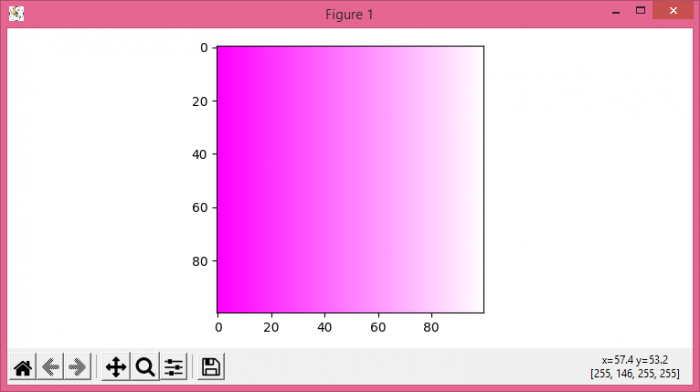# How does imshow handle the alpha channel with an M x N x 4 input?(Matplotlib)

Let's take an example to see how imshow() handles the alpha channel with an M×N×4 input.

## Steps

• Set the figure size and adjust the padding between and around the subplots.
• Return a new array of given shape and type, filled with 1's.
• Handle the alpha channel.
• Display the data as an image, i.e., on a 2D regular raster.
• To display the figure, use show() method.

## Example

import numpy as np
import matplotlib.pyplot as plt

plt.rcParams["figure.figsize"] = [7.50, 3.50]
plt.rcParams["figure.autolayout"] = True

d = np.ones((100, 100, 4), dtype=np.uint8)*255
d[:, :, 1] = np.linspace(0, 255, num=100)

plt.imshow(d)

plt.show()

## Output